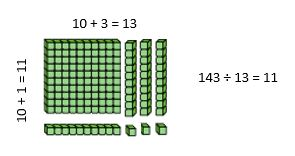# Dividing Using the Area Model in Context of Problem Solving

143 students will be attending the school music concert. Each row will seat 13 students. How many rows will be needed for all of the students to have a seat?Long division. Ugh. When was the last time you used long division to solve a problem as an adult? Most of us estimate and use a mental math strategy or grab a calculator. Why then do we teach this in fifth grade? Students need to know when division is needed to solve a problem and they need to have tools such as mental math to decompose, or break apart, numbers and apply flexible thinking to find the solution.

There are visual tools that help us to see what division is all about. One of those visual models is the area model. You can learn more about the area model through this video that shows visual models of dividing with 2-digit divisors. Video:
Area Model to Divide

Recall our problem: 143 students will be attending the school music concert. Each row will seat 13 students. How many rows will be needed for all of the students to have a seat? The number 143 represents the number of students attending the concert who will sit in the rows.The base ten blocks below are arranged in the area model showing 143 ÷ 13. Since the divisor is 13, the length of one side is 13 units. 143 divided by 13 columns leaves 11 rows. The 13 columns represent the 13 seats in each row. 11 rows are needed to seat all 143 students.By using division problems in the context of a situation, students can make sense of the situation and better understand why division is needed. If division is only taught through straight computation and not in context, it makes it even more challenging for students to make connections and understand the concept of dividing whole numbers. There are many opportunities to engage students in practical situations requiring division.
Here are a few ideas:
• Using area of a garden space at school to determine how many plants can fit in a row
• Calculating how many students can fit in the school cafeteria for an assembly. How many rows will be needed if x students sit in
each row?
• Dividing up funds for a budget shared among class groups
• Planning a family vacation or a class trip
• Dividing up materials needed for a math or science project

To learn more about the area model with division, check out this Learn Zillion video:Learn Zillion Dividing with Area Model